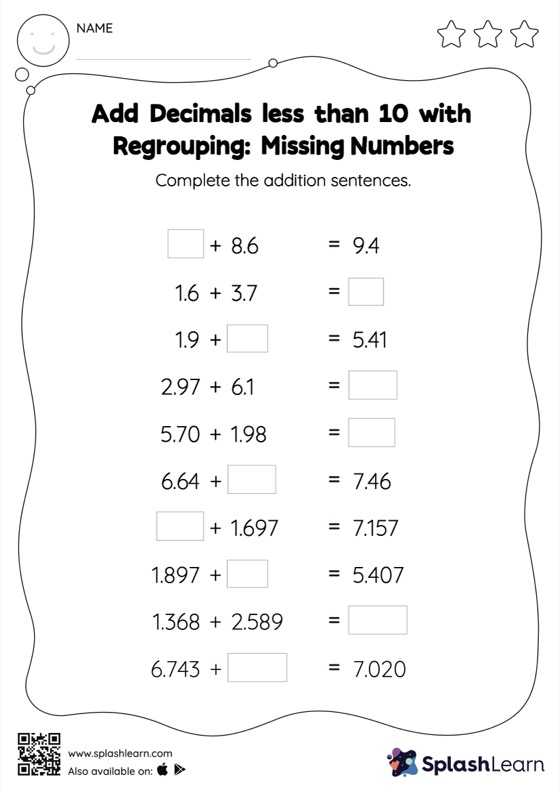# Add Decimals less than 10 with Regrouping: Missing Numbers Worksheet

Home > Add Decimals less than 10 with Regrouping: Missing NumbersLearners hone their math skills with this add decimals less than 10 with regrouping worksheet. While adding decimals, students use the relationship between addition and subtraction to find the missing number. They also need to regroup numbers in add decimals less than 10 with regrouping worksheet utilizing the relationship between ones, tenth, hundredth, thousandth, etc., To get to the result. In each problem, the numbers are laid out in the horizontal format. Students should try to use different strategies involving composing and decomposing numbers to solve these problems. This will help them develop flexibility and fluency.